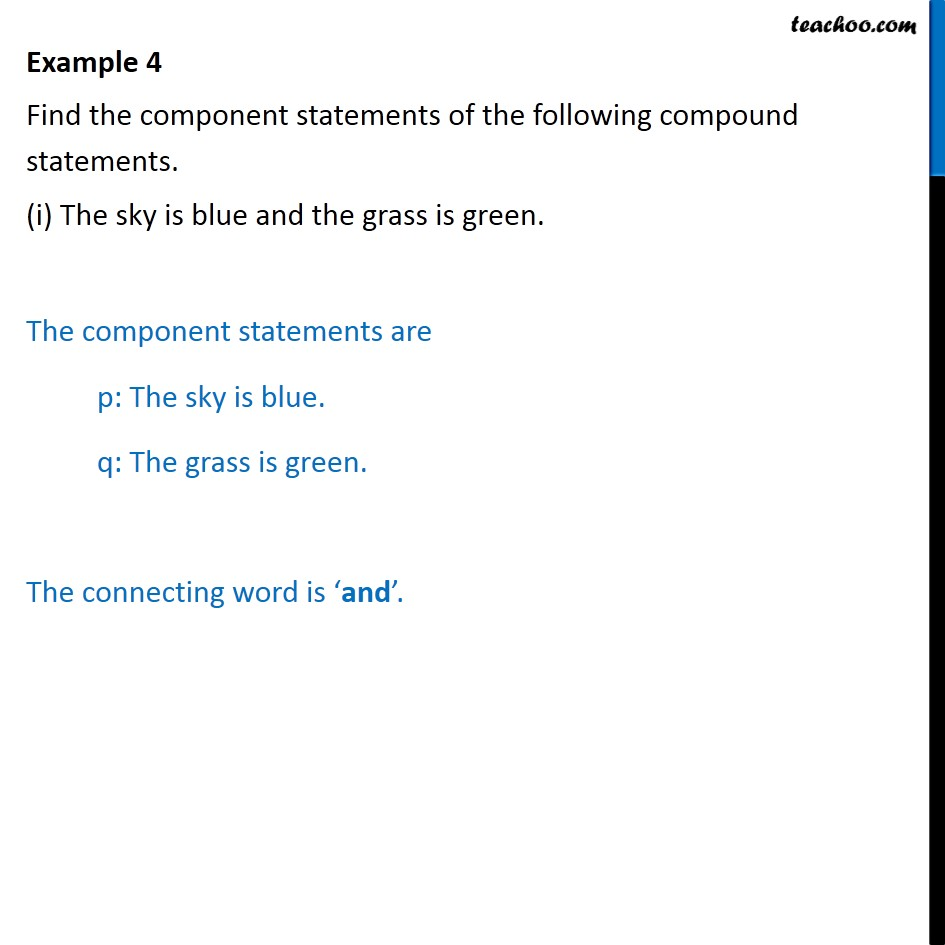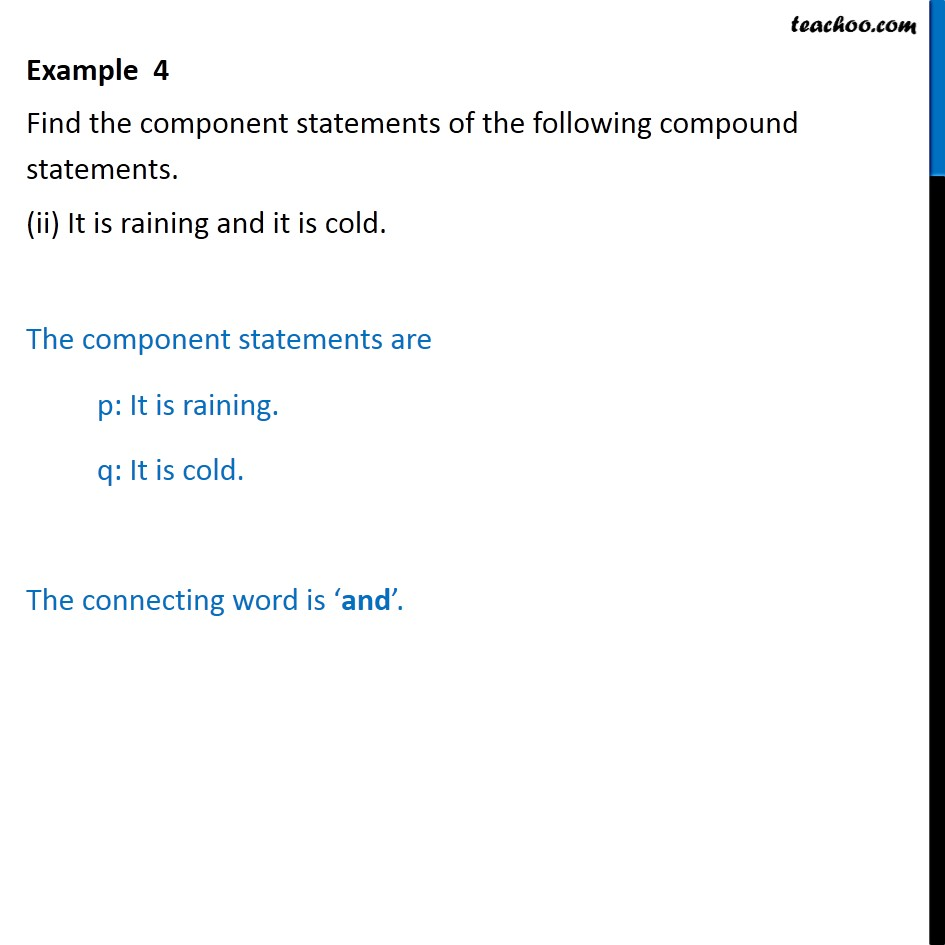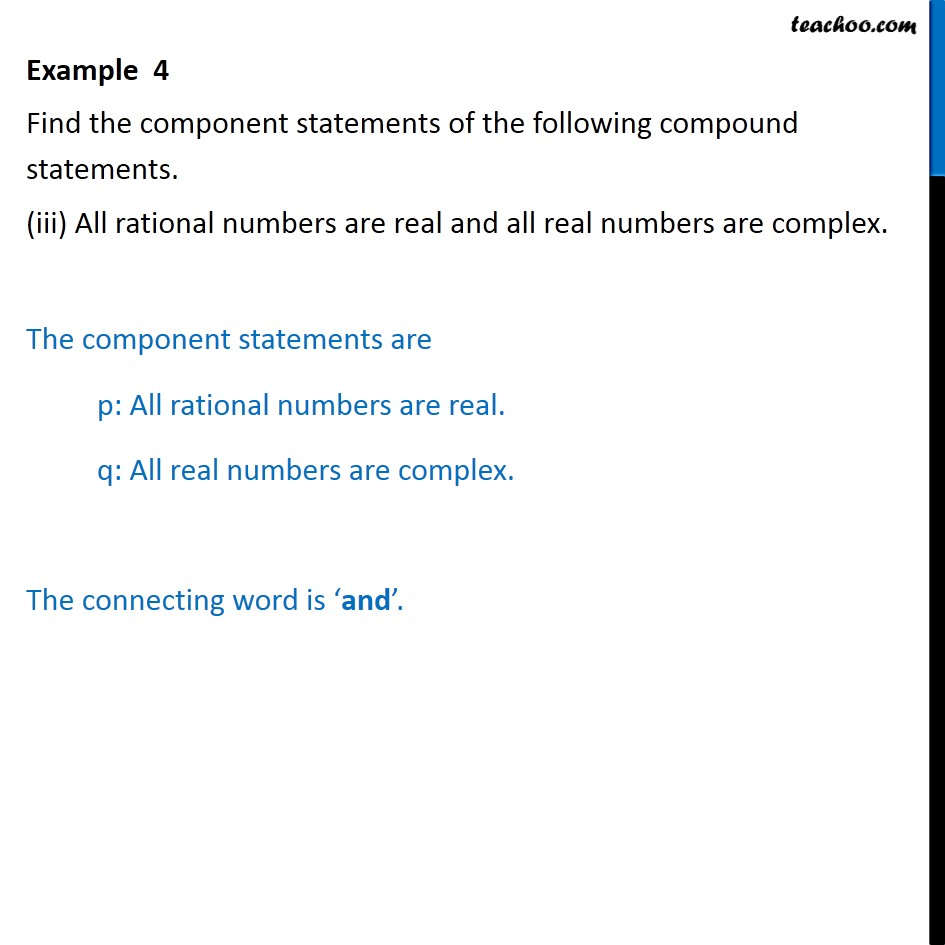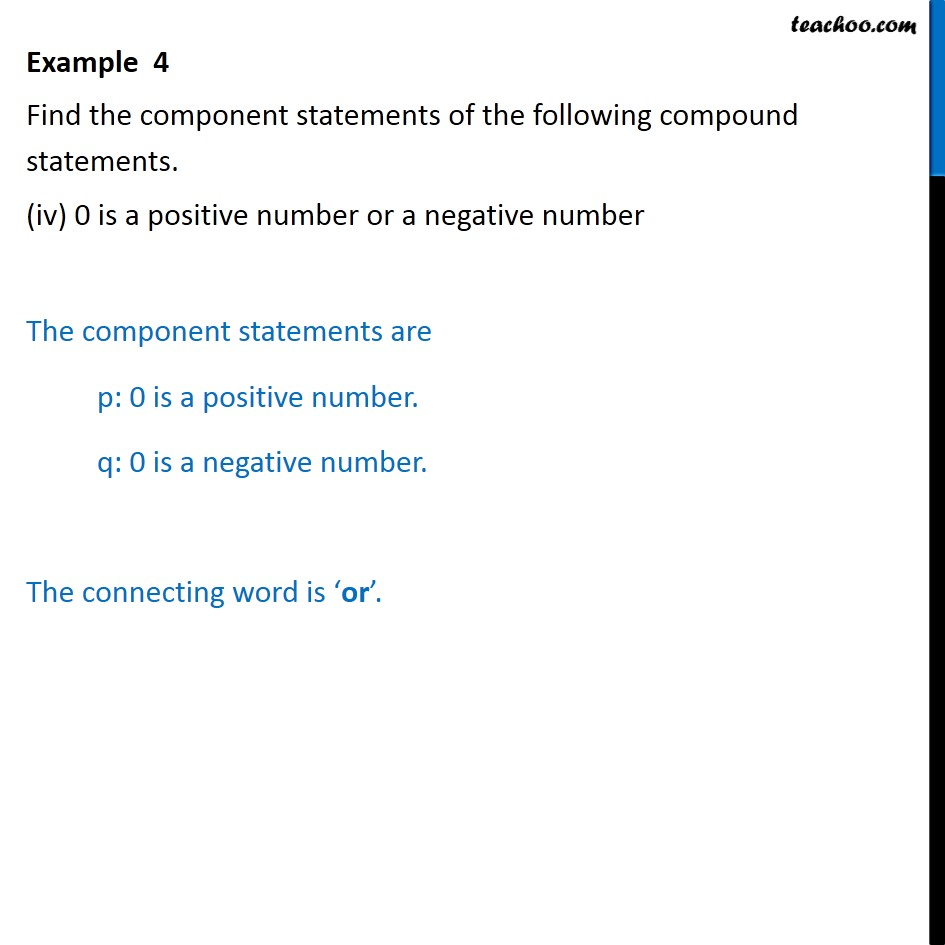Subscribe to our Youtube Channel - https://you.tube/teachoo

1. Chapter 14 Class 11 Mathematical Reasoning
2. Concept wise
3. Finding Compound Statement

Transcript

Example 4 Find the component statements of the following compound statements. (i) The sky is blue and the grass is green. The component statements are p: The sky is blue. q: The grass is green. The connecting word is ‘and’. Example 4 Find the component statements of the following compound statements. (ii) It is raining and it is cold. The component statements are p: It is raining. q: It is cold. The connecting word is ‘and’. Example 4 Find the component statements of the following compound statements. (iii) All rational numbers are real and all real numbers are complex. The component statements are p: All rational numbers are real. q: All real numbers are complex. The connecting word is ‘and’. Example 4 Find the component statements of the following compound statements. (iv) 0 is a positive number or a negative number The component statements are p: 0 is a positive number. q: 0 is a negative number. The connecting word is ‘or’.

Finding Compound Statement